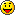SGDK only sub forum

Moderator: Stef

danibus
Very interested
Posts: 63
Joined: Sat Feb 03, 2018 12:41 pm

Just a simple question about sinFix16(value)

I'm working with a sprite with horizontal movement, always to the left, then this sprite will disappear from left side of screen and then appear on the right side... and so. I wanted a sin movement, then made:

y = 84 + sinFix16(x); // 84 is the initial 'y' position of the sprite

sinFix16(x) goes from 64 to -64, so Y = 20 .. 148

Why 64 to -64 ???? I read that sinFix16(value) needs a value from 0 to 1024, I just put 'x' and works... not sure whyI just working with int
If I want to a better sin movement, use fix32 or fix16?

danibus
Very interested
Posts: 63
Joined: Sat Feb 03, 2018 12:41 pm

### Re: About sinFix16()

Code: Select all

``````static void moveEnemy()
{
int vmovx = 1;

enemyPosx -= vmovx ;		// move to the left, enemyPosx  and enemyPosy    are INTs
enemyPosx = contsY + sinFix16(enemyPosx);   // contsY =84;

SPR_setPosition(enemy, enemyPosx, enemyPosy);

}
``````
This is my function to move enemy sprite with sinusoidal movement.
I want sprite to move up/down across the screen, but it moves to slow to do it only in one screen.

ThisI tried

Code: Select all

``enemyPosx = contsY + sinFix16(enemyPosx) * x;  // x = 1.25   or  2``
but also to fast going up/down

Maybe using INTs for (x,y) is the problem

Chilly Willy
Very interested
Posts: 2768
Joined: Fri Aug 17, 2007 9:33 pm

### Re: About sinFix16()

danibus wrote:
Fri Aug 02, 2019 3:42 am
Why 64 to -64 ???? I read that sinFix16(value) needs a value from 0 to 1024, I just put 'x' and works... not sure whyIt goes from 64 to -64 because with the fixed point 16-bit numbers in SGDK, that's 1 to -1. Remember, the fractional part of the fix16 is 6 bits. The table goes from 0 to 1024 because it's a binary angle. Binary angles use convenient powers of two so that you can use AND to clamp the range to the table. Radians are not so good a unit for a processor like the 68000.1024 entries gives a sufficiently smooth sine wave without taking too much rom space. Especially considering the limits of the fractional parts of fixed point numbers (fix16 in particular).

Stef
Very interested
Posts: 2976
Joined: Thu Nov 30, 2006 9:46 pm
Location: France - Sevres
Contact:

### Re: About sinFix16()

Chilly Willy perfectly explained the reason of the value you obtained and sin table sizeIf you want faster sin wav depending x position, try something as :

Code: Select all

``enemyPosy = contsY + sinFix16(enemyPosx * sinSpeed);``
If you also want larger sin amplitude then multiply that way :

Code: Select all

``enemyPosy = contsY + sinFix16(enemyPosx * sinSpeed) * Amplitude;``

danibus
Very interested
Posts: 63
Joined: Sat Feb 03, 2018 12:41 pm

### Re: About sinFix16()

Thanks for replying. Not sure I understand hehe

2 ^ 6 = 64 (fractional part of the fix16 is 6 bits)

So let's check

Code: Select all

``````...
int enemyPosx = 260;  //starter position
...
int sinSpeed = 2;
int Amplitude = 1;
enemyPosx = enemyPosx - 1;   //moves 1 pixel (60 pixels/sec aprox)
enemyPosy = contsY + sinFix16(enemyPosx * sinSpeed) * Amplitude;
...
``````
Enemy is moving from right to left:

enemyPosy = 84 + sinFix16(260 * 2) * 1 = 84 + sinFix16(520) = 81 ( then sinFix16(520) = -3 )
enemyPosy = 84 + sinFix16(259 * 2) * 1 = 84 + sinFix16(518) = 82 ( then sinFix16(518) = -2 )
enemyPosy = 84 + sinFix16(258 * 2) * 1 = 84 + sinFix16(516) = 82 ( then sinFix16(516) = -2 )
enemyPosy = 84 + sinFix16(257 * 2) * 1 = 84 + sinFix16(514) = 83 ( then sinFix16(514) = -1 )
enemyPosy = 84 + sinFix16(256 * 2) * 1 = 84 + sinFix16(512) = 84 ( then sinFix16(512) = 0 )
...

SINE WAVE table have 1024 entries ( varies 2 every time):
1024 -> 0 ---> that means sinFix16(1024) = 0
1022 -> -1
1020 -> -2
1018 -> -2
1016 -> -3
...
768 -> -64
...
516 -> -2
514 -> -1
512 -> 0
510 -> 1
508 -> 2
...
2 -> 1
0 -> 0

That means starts with negative values (SIN wave starts moving down... then goes up to 0, goes up and goes down again)
from 0 to -64, from -64 to 0 to 64, again from 64 to 0

IF Amplitude = 2, then sinFix16() will go from 0 to -128, from -128 to 0 to 128, again from 128 to 0
And so

Chilly Willy
Very interested
Posts: 2768
Joined: Fri Aug 17, 2007 9:33 pm

### Re: About sinFix16()

The table starts at 0 and goes to 1023. 0 binary angle units is the same as 0 degrees, 256 is the same as 90 degrees, 512 is the same as 180 degrees, 768 is the same as 270 degrees, and 1024 would be the same as 360 degrees. You AND the binary angle you are using with 1023 (0x3FF) so that anything more than 360 wraps to 0 since the sin repeats every 360 degrees (every 1024 binary angle units in this case). You can use the table backwards, but then you have the negative of the sin wave.

If you check the source, the cos function is simply sinFix16[(x + 256) & 1023] because the cos leads the sin by 90 degrees (256 binary angle units).